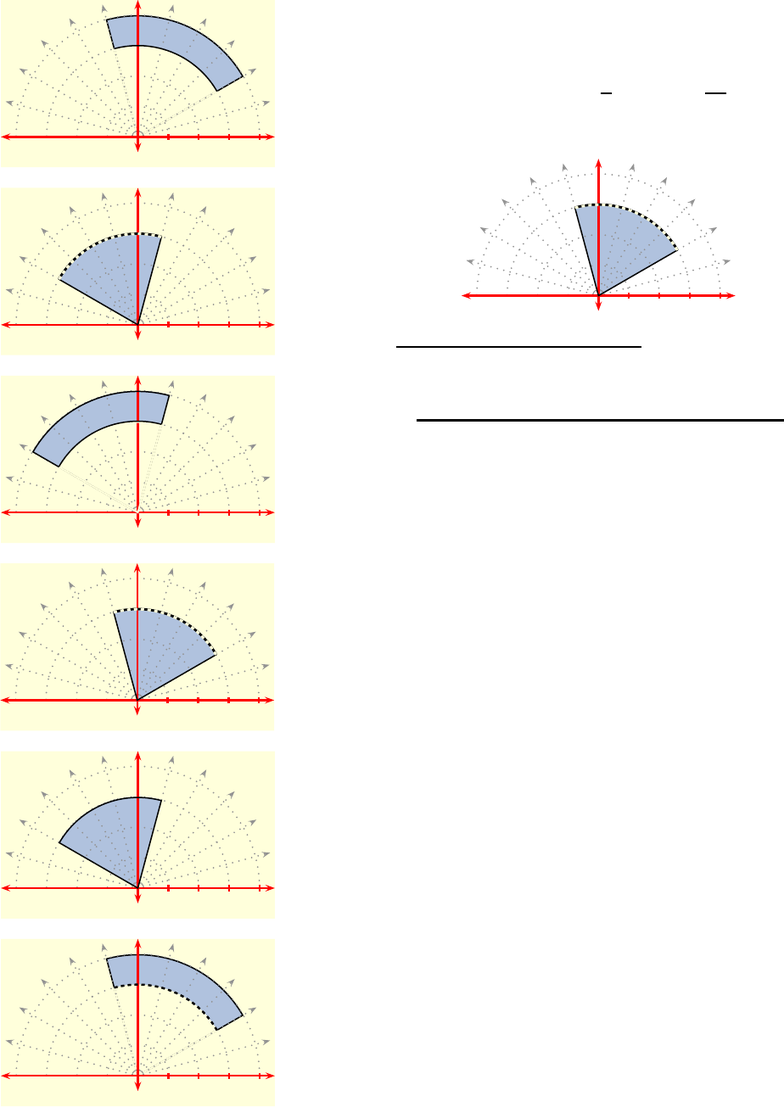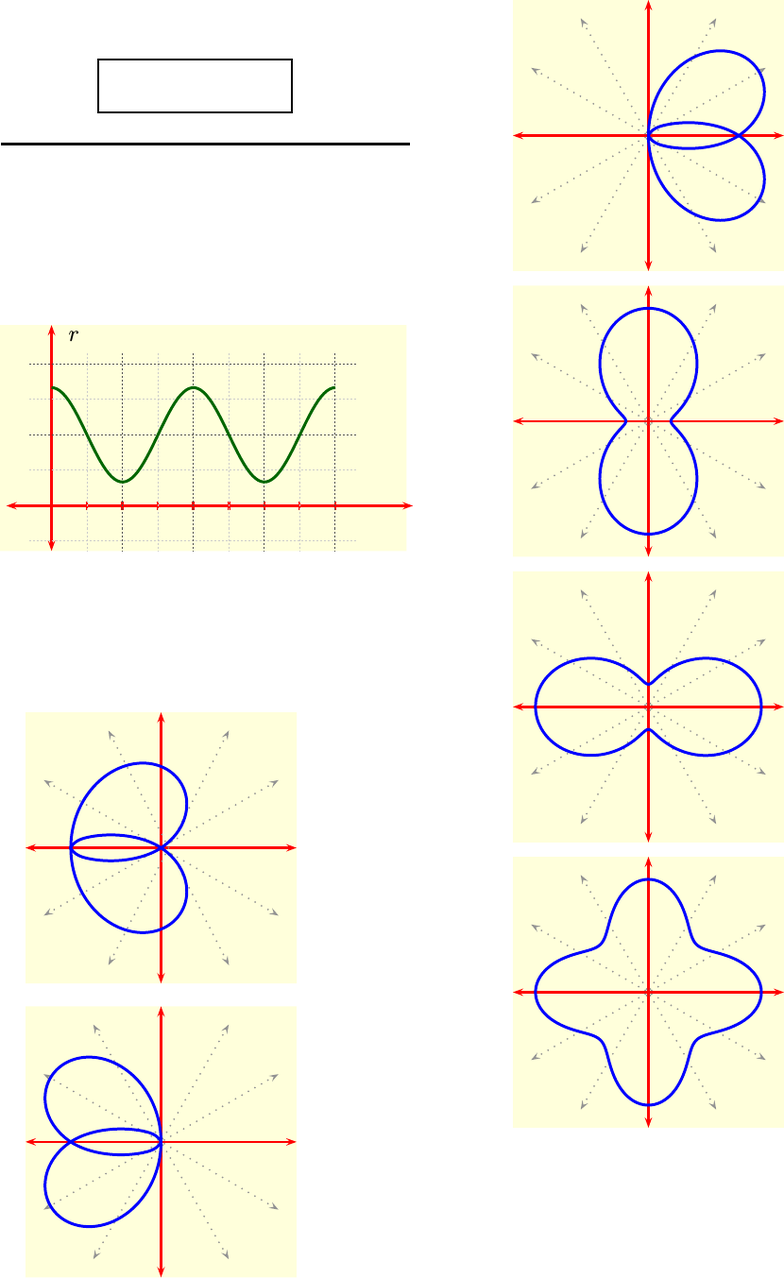# M 408D Lecture 2: HW 02-solutions

60 views13 pages
School
UT-Austin
Department
Mathematics
Course
M 408D
Professoroliver (smo998) – HW 02 – rusin – (52850) 1
This print-out should have 20 questions.
Multiple-choice questions may continue on
the next column or page – ﬁnd all choices
001 10.0 points
If ais a vector parallel to the xy-plane and
bis a vector parallel to k, determine ka×bk
when kak= 3 and kbk= 2.
1. ka×bk= 0
2. ka×bk=3
3. ka×bk= 3
4. ka×bk= 6 correct
5. ka×bk=6
6. ka×bk=32
7. ka×bk= 32
Explanation:
For vectors aand b,
ka×bk=kakkbksin θ
when the angle between them is θ, 0 θ < π.
But θ=π/2 in the case when ais parallel
to the xy-plane and bis parallel to kbe-
cause kis then perpendicular to the xy-plane.
Consequently, for the given vectors,
ka×bk= 6 .
keywords: cross product, length, angle,
002 10.0 points
A line passes through the point P(2,3,3)
and is perpendicular to the plane
x+ 4y+ 3z= 6 .
At what point Qdoes intersect the xy-
plane?
1. Q(3,7,0)
2. Q(3,0,1)
3. Q(1,1,0) correct
4. Q(7,0,3)
5. Q(1,0,7)
6. Q(1,1,0)
Explanation:
Since the xy-plane is given by z= 0, we
have to ﬁnd an equation for and then set
z= 0.
Now a line passing through a point
P(a, b, c) and having direction vector vis
given parametrically by
r(t) = a+tv,a=ha, b, c i.
But for , its direction vector will be parallel
to the normal to the plane
x+ 4y+ 3z= 6 .
Thus
a=h2,3,3i,v=h1,4,3i,
and so
r(t) = h2 + t, 3 + 4t, 3 + 3ti.
In this case, z= 0 when t=1. Conse-
quently, intersects the xy-plane at
Q(1,1,0) .
keywords: line, parametric equations, direc-
tion vector, point on line, intercept, coordi-
nate plane
003 10.0 points
Which one of the following shaded-regions
in the plane consists of all points whose polar
coordinates satisfy the inequalities
0r < 3,1
6πθ7
12π?
Unlock document

This preview shows pages 1-3 of the document.
Unlock all 13 pages and 3 million more documents.oliver (smo998) – HW 02 – rusin – (52850) 2
1.
2 4
2.
2 4
3.
2 4
4.
2 4
correct
5.
2 4
6.
2 4
Explanation:
Using the deﬁnition of polar coordinates
(r, θ), we see that the region deﬁned by the
inequalities
0r < 3,1
6πθ7
12π
is
2 4
keywords: polar coordinates, inequalities, po-
lar graph,
004 10.0 points
Find a polar equation for the curve given
by the Cartesian equation
4y2=x .
1. r= 4 csc θtan θ
2. 4r= sec θtan θ
3. 4r= sec θcot θ
4. 4r= csc θcot θcorrect
5. r= 4 csc θcot θ
6. r= 4 sec θtan θ
Explanation:
We have to substitute for x, y in
4y2=x
using the relations
x=rcos θ , y =rsin θ .
In this case the Cartesian equation becomes
4r2sin2θ=rcos θ .
Unlock document

This preview shows pages 1-3 of the document.
Unlock all 13 pages and 3 million more documents.oliver (smo998) – HW 02 – rusin – (52850) 3
Consequently, the polar form of the equation
is
4r= csc θcot θ .
005 10.0 points
Use the graph in Cartesian coordinates
r
θ
π2π
of ras a function of θto determine which
one of the following is the graph of the corre-
sponding polar function?
1.
2.
3.
4.
5. correct
6.
Explanation:
As the Cartesian graph of rnever crosses
the θ-axis, there is no value of θin [0,2π]
at which r= 0; in particular, therefore, the
polar graph never passes through the origin.
This eliminates 3 of the graphs, leaving only
Unlock document

This preview shows pages 1-3 of the document.
Unlock all 13 pages and 3 million more documents.